# Modelling

In order to predict the concentration of different substance in E.coli, we set the kinetic model according to the reaction rate theory and enzymatic reaction kinetics as our first model in our project. And the production and conversion rate model is included to simulate the directed evolution model and the natural evolution model, and then we can get the time we need in our directed evolution method of DTE. The third model we set up is the microfluidics model to predict and simulate the situation in the microfluidics chip, which is our hardware for the gradient concentration of the psicose and antibiotic to let us have a high throughput experiment. The fourth model we set is the market prediction model to predict the future market and the coefficient between different age groups and the tendency to adopt psicose.

1. Psicose Synthesis Kinetic Model
2.
4.
5. Market Prediction Model
6.
7. Hairpin Coupling Model

### The Establishment of Psicose Synthesis Kinetic Model

In our design, the DTE process is one of the most significant parts in manufacturing psicose. The main process of psicose manufacture is catalyzed by D-psicose 3-epimerase. The models of device A, B, C and D are as follows.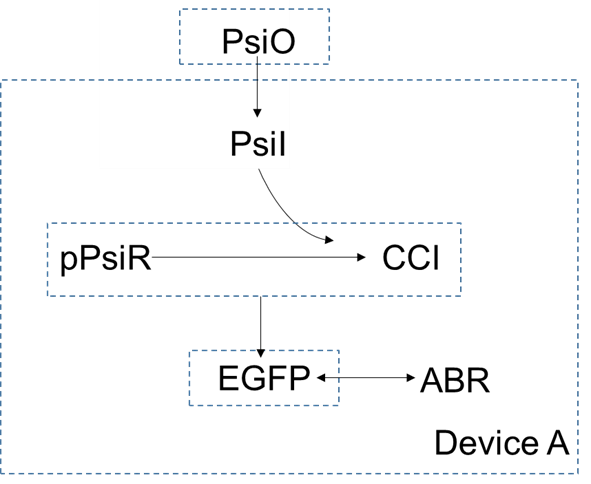In device A, extracellular concentration of $psicose$ is higher than the intracellular concentration, so it can enter the cells by diffusion. As a small molecular, the $psicose$ inside the cell can be combined with $pPsiR$ to generate $CCI$. $pPsiR$ is a repressor, which can bind to promoters on DNA and block gene expression. After binding with $psicode$,$pPsiR$ falls off from the promoter and the gene starts expressing, And eventually produce the produce $EGFP$.

For device A, the dynamic equations can be listed as follows:

Concentrations of $psicose$ are different inside and outside the cell, so the diffusion rate of $psicose$ is proportional to the concentration difference between inside and outside of the cells. so the rate at which $psicose$ enters the cell by diffusion is $$-\gamma_F([PsiO]-[PsiI])$$
Where $\gamma_F$ is the diffusion coefficient.

Considering that the volume of the cells is small enough to be negligible relative to the external solution, so the amount of $psicose$ that diffuses into the cells is very small. We can think of the concentration of the external solution as constant, which means $$\frac{\text{d}[PsiO]}{ \text{d}t}=0$$ When reducer psicose combines with repressor, the process is $$\eta_1 PsiI+pPsiR\rightarrow CCI$$ Where $PsiI$ is the intracellular $psicose$, $pPsiR$ is $psicose$ dependent repressor, $CCI$ is $psicose$-repressor complex and $\eta_1$ is coordination number of $psicose$ for $pPsiR$.

The change in concentration of $PsiI$，$pPsiR$ and $CCI$ contains three influencing factors: the binding reaction of $pPsiR$ and $psicose$, and the degradation reaction of themselves and the reverse reaction.

Here we consider the binding reaction as a second order reaction and the degradation reaction and reverse reaction as first order reactions. According to law of mass action, reaction rate is proportional to the product of the reactant concentration, so we have $$\frac{\text{d}[PsiI]}{\text{d}t}=-\gamma_F([PsiI]-[PsiO])-m_{pPsiR,Psi}[PsiI]^{\eta_1}[pPsiR]+m_{CCI}[CCI]-\delta_{PsiI}[PsiI]$$ And considering $pPsiR$ is constantly expressing, we can get $$\frac{\text{d}[pPsiR]}{\text{d}t}=\alpha_{pPsiR}-m_{pPsiR,Psi}[PsiI]^{\eta_1}[pPsiR]+m_{CCI}[CCI]-\delta_{pPsiR}[pPsiR]$$ Where $m_{pPsiR,Psi}$ is the coefficient of reaction rate of the binding reaction, $\alpha_{pPsiR}$ is the rate of constant expression of $pPsiR$, $\delta_{pPsiR}$ and $\delta_{PsiI}$ are coefficients of reaction rate of the degradation reaction of $pPsiR$ and $PsicoseI$ respectively.
The concentration of inactivated repressor is $$\frac{\text{d}[CCI]}{\text{d}t}=m_{pPsiR,Psi}[PsiI]^{\eta_1}[pPsiR]-m_{CCI}[CCI]-\delta_{CCI}[CCI]$$ The change in $EGFP$ concentration depends on the concentration of its repressor, $pPsiR$ which can be described by Hill-equation. Considering $EGFP$ is also degrading, the equation is $$\frac{\text{d}[EGFP]}{\text{d}t}=H\frac{\beta_{EGFP}K^n}{K^n+[pPsiR]^n} -\delta_{EGFP}[EGFP]$$ Where $n$ is hill coefficient, $K$ is the ligand concentration producing half occupation, $\beta_{EGFP}$ is maximal transcription rate of gene $EGFP$, and $H$ is a constant used to indicate the deviation between the theoretical and actual values.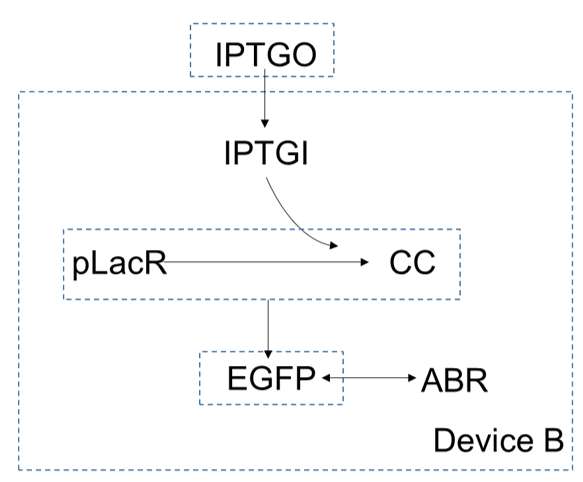In device B, extracellular concentration of $IPTG$ is higher than which is intracellular, so it can enter the cells by diffusion. As a small molecular, the $IPTG$ inside the cell can be combined with $pLacR$ to generate $CC$. $pLacR$ is a repressor, which can bind to promoters on DNA and block gene expression. After binding with $IPTG$, $pLacR$ falls off from the promoter and the gene starts expressing, and eventually produce the produce $EGFP$. Since the gene of $ABR$ and the gene of $EGFP$ are connected in series, they are expressed together.

Similarly, we can get the function of device B by using the reaction rate equation and the diffusion function. First, $$\frac{\text{d}[IPTGO]}{\text{d}t}=0$$ According to the reaction between $IPTGI$ and $pLacR$ and the reaction rate equation, we can get $$\eta_2 IPTGI+pLacR\rightarrow CC$$ Where $\eta_2$ is coordination number of $IPTG$ for $pLacR$.

This reaction is similar with the reaction in device A, so we have $$\frac{\text{d}[IPTGI]}{\text{d}t}=-\gamma_{IPTG}([IPTGI]-[IPTGO])-m_{IPTG,pLacR}[IPTGI]^{\eta_2}[pLacR]+m_{CC}[CC]-\delta_{IPTG}[IPTGI]$$ Similarly, we can also get $$\frac{\text{d}[pLacR]}{\text{d}t}=\alpha_{pLacR}-m_{IPTG,pLacR}[IPTGI]^{\eta_2}[pLacR]+m_{CC}[CC]-\delta_{pLacR}[pLacR]$$ $$\frac{\text{d}[CC]}{\text{d}t}=m_{IPTG,pLacR}[IPTGI]^{\eta_2}[pLacR]-m_{CC}[CC]-\delta_{CC}[CC]$$ And $pLac$ is a transcription activator of gene $EGFP$, according to hill equation, the concentration of $EGFP$ is $$\frac{\text{d}[EGFP]}{\text{d}t}=H\frac{\beta_{EGFP}K^n}{K^n+[pLacR]^n} -\delta_{EGFP} [EGFP]$$ The concentration of $EGFP$ is the same as the concentration of antibiotics resistance due to the transcription and translation of antibiotic resistance gene combined with the gene of $EGFP$. $$\frac{\text{d}[EGFP]}{\text{d}t}=\frac{\text{d}[ABR]}{\text{d}t}$$ Where $[EGFP]$ is the concentration of $EGFP$ and $[ABR]$ is the concentration of antibiotic resistance protein expression.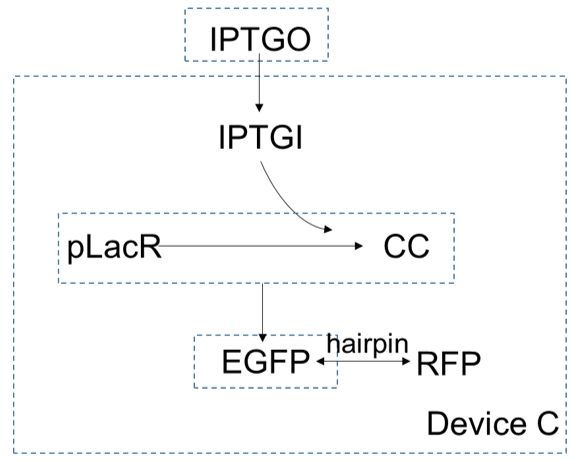Device C is the same with device B. The only difference is the hairpin between gene of $EGFP$ and gene of $RFP$. Similarly, we can get the function of hairpin and its coefficient.

The first few equations are the same as in device B: $$\frac{\text{d}[IPTGO]}{\text{d}t}=0$$ $$\frac{\text{d}[IPTGI]}{\text{d}t}=-\gamma_{IPTG}([IPTGI]-[IPTGO])-m_{IPTG,pLacR}[IPTGI][pLacR]+m_{CC}[CC]-\delta_{IPTG}[IPTGI]$$ $$\frac{\text{d}[pLacR]}{\text{d}t}=\alpha_{pLacR}-m_{IPTG,pLacR}[IPTGI][pLacR]+m_{CC}[CC]-\delta_{pLacR}[pLacR]$$ $$\frac{\text{d}[CC]}{\text{d}t}=m_{IPTG,pLacR}[IPTGI][pLacR]-m_{CC}[CC]-\delta_{CC}[CC]$$ $$\frac{\text{d}[EGFP]}{\text{d}t}=H\frac{\beta_{EGFP}K^n}{K^n+[pLacR]^n} -\delta_{EGFP} [EGFP]$$ The presence of the hairpin leads to a decrease in $RFP$ expression efficiency, so we have $$\frac{\text{d}[EGFP]}{\text{d}t}=k\frac{\text{d}[RFP]}{\text{d}t}$$ Where $[RFP]$ is the concentration of red fluorescence protein, $k$ is the coefficient of hairpin.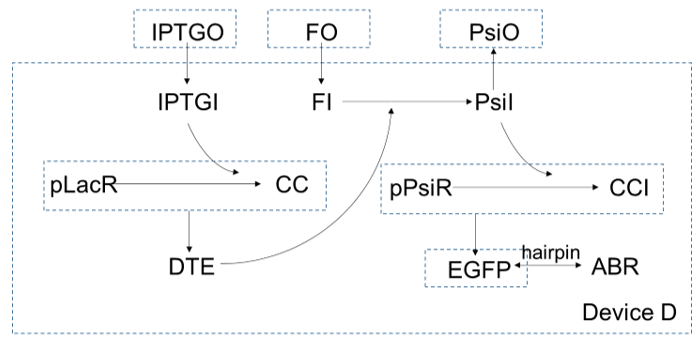In device D, $IPTG$ gets in cells and bind with $pLacR$, which is a repressor for gene $DTE$. After $IPTG$ binding with $pLacR$, $DTE$ starts to express, and as an enzyme, to catalysis $fructose$ to turn into $psicose$. As more and more $psicose$ are produced, more and more the repressor of gene $EGFP$, $pLacR$ are inactivated, so expression of $EGPF$ increase. At the same time, expression of $ABR$ also increase since the gene of $ABR$ and the gene of $EGFP$ are connected in series by a hairpin.

For device D, $psicose$ and $fuctose$ get in cells by diffusion: $$\frac{\text{d}[PsiO]}{\text{d}t}=\frac{-\gamma_{F}([PsiO]-[PsiI])}{V_{outside}}=0$$ $$\frac{\text{d}[FO]}{\text{d}t}=\frac{-\gamma_{F}([FO]-[FI])}{V_{outside}}=0$$ $$\frac{\text{d}[IPTGO]}{\text{d}t}=\frac{-\gamma_{F}([IPTGO]-[IPTGI])}{V_{outside}}=0$$ And device D consists of device A and device C connected by an extra step:

$$FI\overset{DTE}{\rightarrow}PsiI$$ This reaction is an enzyme catalyzed reaction, which can be described by Michaelis equation, so we can get the following equations: $$\frac{\text{d}[FI]}{\text{d}t}=\gamma_{F}([FI]-[FO])-\frac{k_2[DTE][FI]}{K_M+[FI]}-\delta_{FI}[FI]$$ $$\frac{\text{d}[PsiI]}{\text{d}t}=-\gamma_{F}([PsiI]-[PsiO])+\frac{k_2[DTE][FI]}{K_M+[FI]}-\delta_{PsiI}[PsiI]$$ $$\frac{\text{d}[IPTGI]}{\text{d}t}=-\gamma_{IPTG}([IPTGI]-[IPTGO])+m_{IPTG,pLacR}[IPTGI][pLacR]-\delta_{IPTG}[IPTGI]$$ Where $k_2$ is reaction rate coefficient of transition state product’s decomposition reaction, $K_M$ is the Michaelis contant.
The rest of the equations are the same with which in device A and device C: $$\frac{\text{d}[pLacR]}{\text{d}t}=\alpha_{pLacR}-m_{IPTG,pLacR}[IPTGI][pLacR]+m_{CC}[CC]-\delta_{pLacR}[pLacR]$$ $$\frac{\text{d}[pPsiR]}{\text{d}t}=\alpha_{pPsiR}-m_{pPsiR,PsiI}[pPsiR][PsiI]+m_{CCI}[CCI]-\delta_{pLacR}[pLacR]$$ $$\frac{\text{d}[pPsiR]}{\text{d}t}=\alpha_{pPsiR}-m_{pPsiR,Psi}[PsiI][pPsiR]+m_{CCI}[CCI]-\delta_{pPsiR}[pPsiR]$$ $$\frac{\text{d}[CC]}{\text{d} t}=m_{IPTG,pLacR}[PsiI][pPsiR]-m_{CC}[CC]-\delta_{CC}[CC]$$ $$\frac{\text{d}[CCI]}{\text{d} t}=m_{pSiR}[PsiI][pPsiR]-m_{CCI}[CCI]-\delta_{CCI}[CCI]$$ $$\frac{\text{d}[DTE]}{\text{d}t}=H\frac{\beta_{EGFP}K^n}{K^n+[pLacR]^n}-\delta_{DTE}[DTE]$$ $$\frac{\text{d}[EGFP]}{\text{d}t}=H\frac{\beta_{EGFP}K^n}{K^n+[pPsiR]^n}-\delta_{EGFP}[EGFP]$$ $$\frac{\text{d}[ABR]}{\text{d}t}=k\frac{\text{d}[EGFP]}{\text{d}t}$$ The results are shown in the Results Tab as follows.

### Device A Validation

To validate our ODE model, we cooperate with SYSU and share our wetLab data to validate our model and their software CO-RAD. This year, SYSU-Software developed CO-RAD, a Collaborative Optimization platform with multiple functions including Recommendation, Analysis and Design. During the conversion with them, they offered to build ODE system to predict the behavior of device, which can verify our design from another perspective. They built the model with their software CO-RAD, based on our circuit design device A: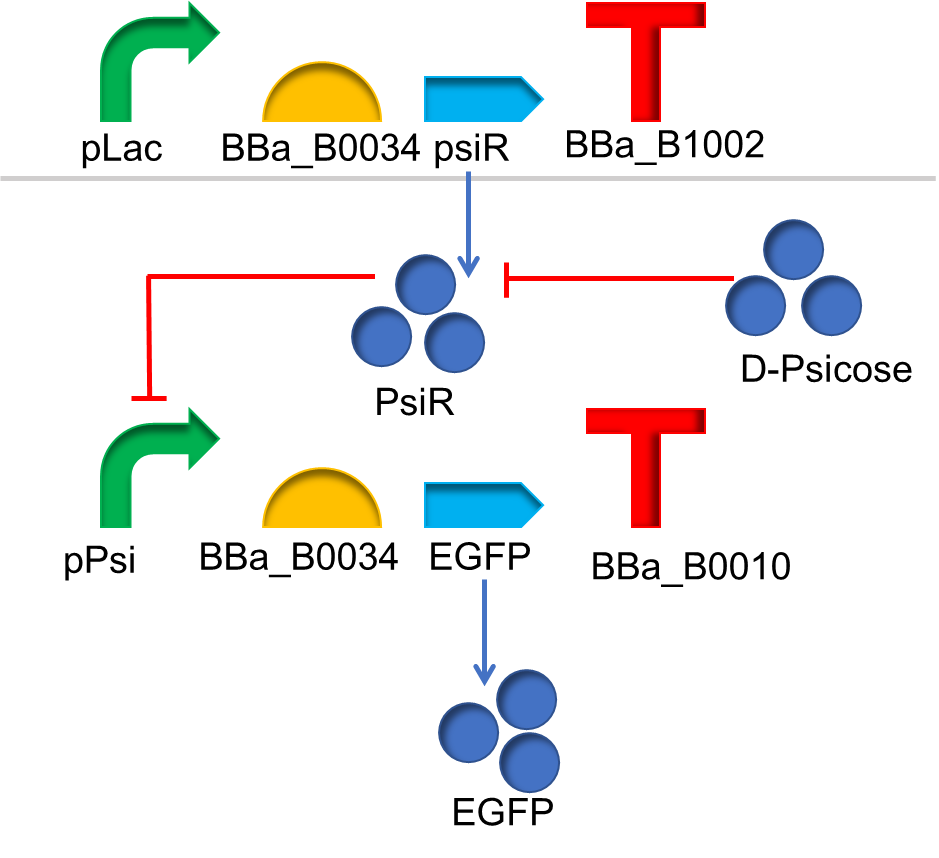In this genetic circuit, PsiR is a predicted LacI family transcription factor with high affinity for D-Psicose. This implies that PsiR is potentially capable of binding a consensus sequence in the promoter region and preventing transcription of the regulated promoters in the absence of D-Psicose. With addition of D-psicose, EGFP can be successfully produced. With their visual designer and the embedded mathematical ODE systems model, they constructed our device A and dynamically analyzed the amounts of materials in it. They pay attention to the expression of EGFP, since its amount can be evaluated in unit of optical density. Related formulas and parameters are shown below: $$\frac{d[PsiR]}{dt} = \frac{k_{PsiR}[gene_{PsiR}]}{1+[Psicose]^{n1}}-d_{PsiR}[PsiR]$$ $$\frac{d[EGFP]}{dt}=\frac{k_{EGFP}[gene_{EGFP}]}{1+[PsiR]^{n2}}-d_{PsiR}[EGFP]$$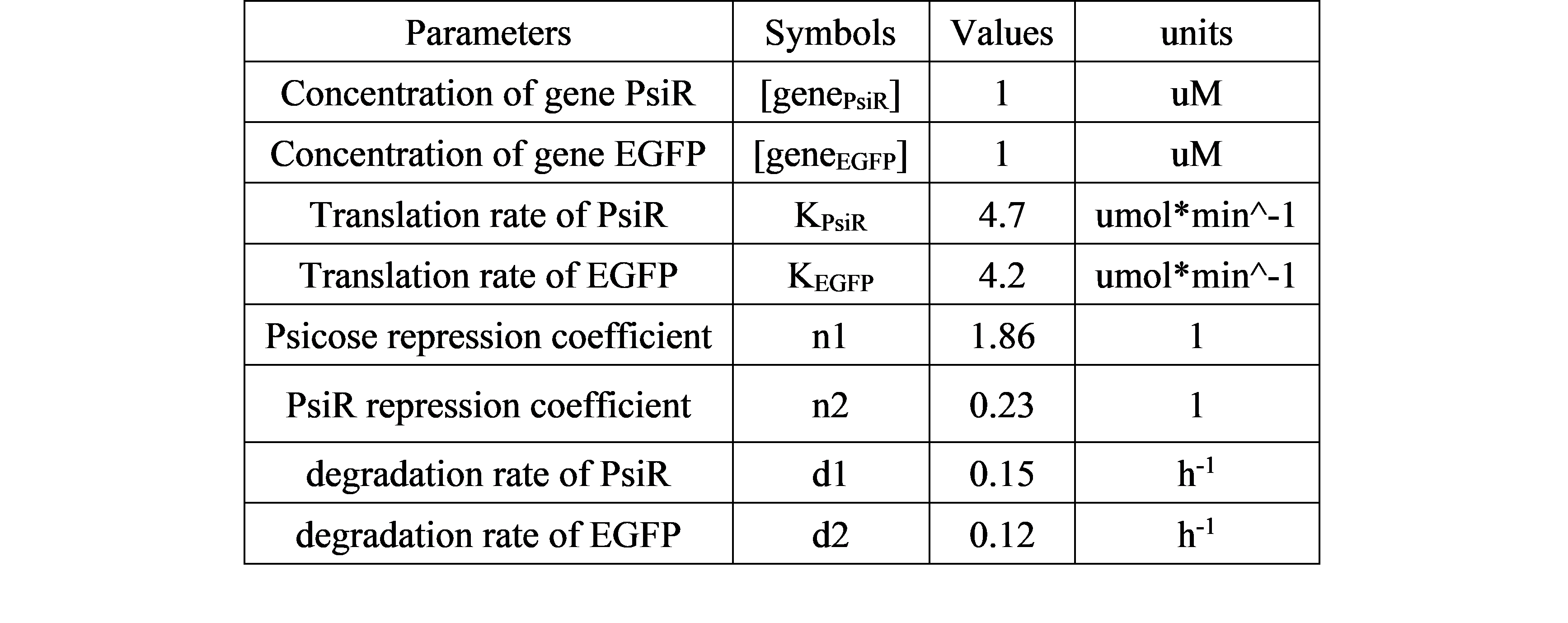Basing our model, we can calculate and simulate our system as follows: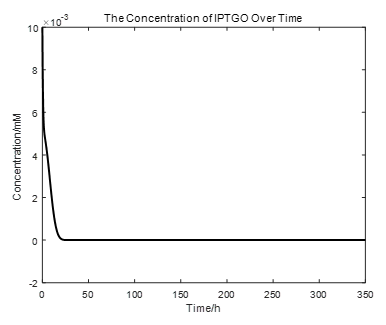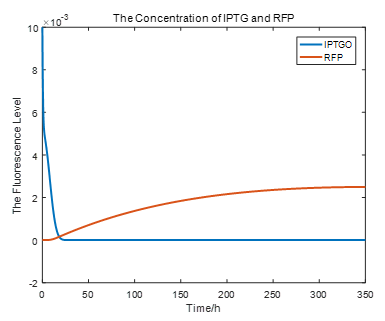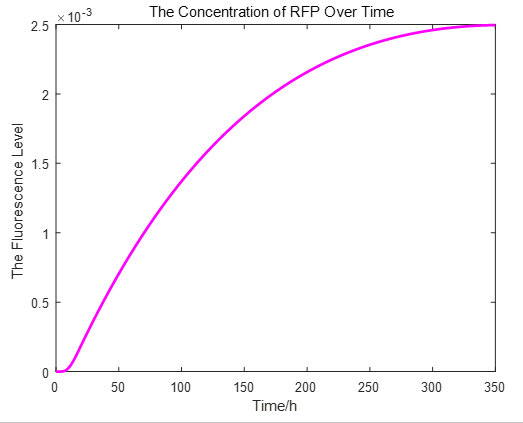From the picture below, it is evident that with time going by, the concentration of IPTG outside the cell will decrease due to the diffusion process, while the RFP expression level is increasing stably.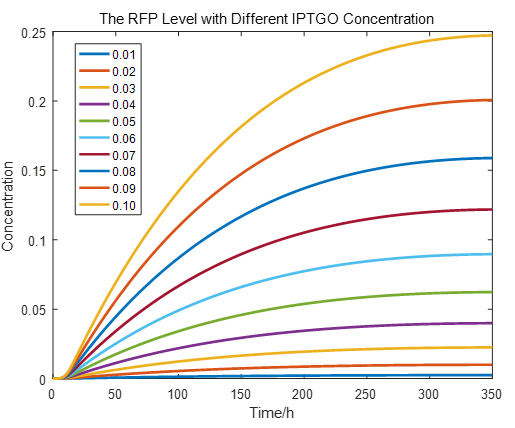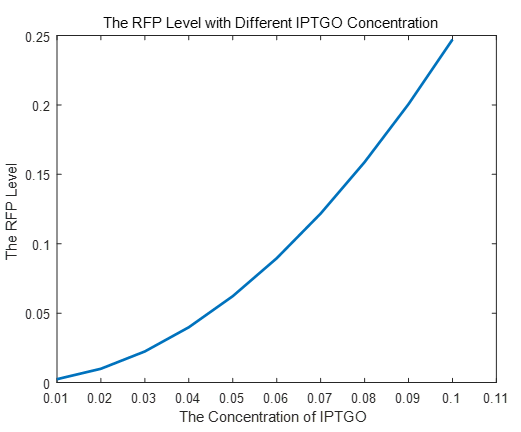If we do more experiments shown below, we can find that the expression level of RFP is increasing with the increase of the concentration of IPTG, which proves that we can get a linear range of IPTG to get the RFP expression level. According to device A, B, C, D, this conclusion can also be made, which proves the validation of our kinetic model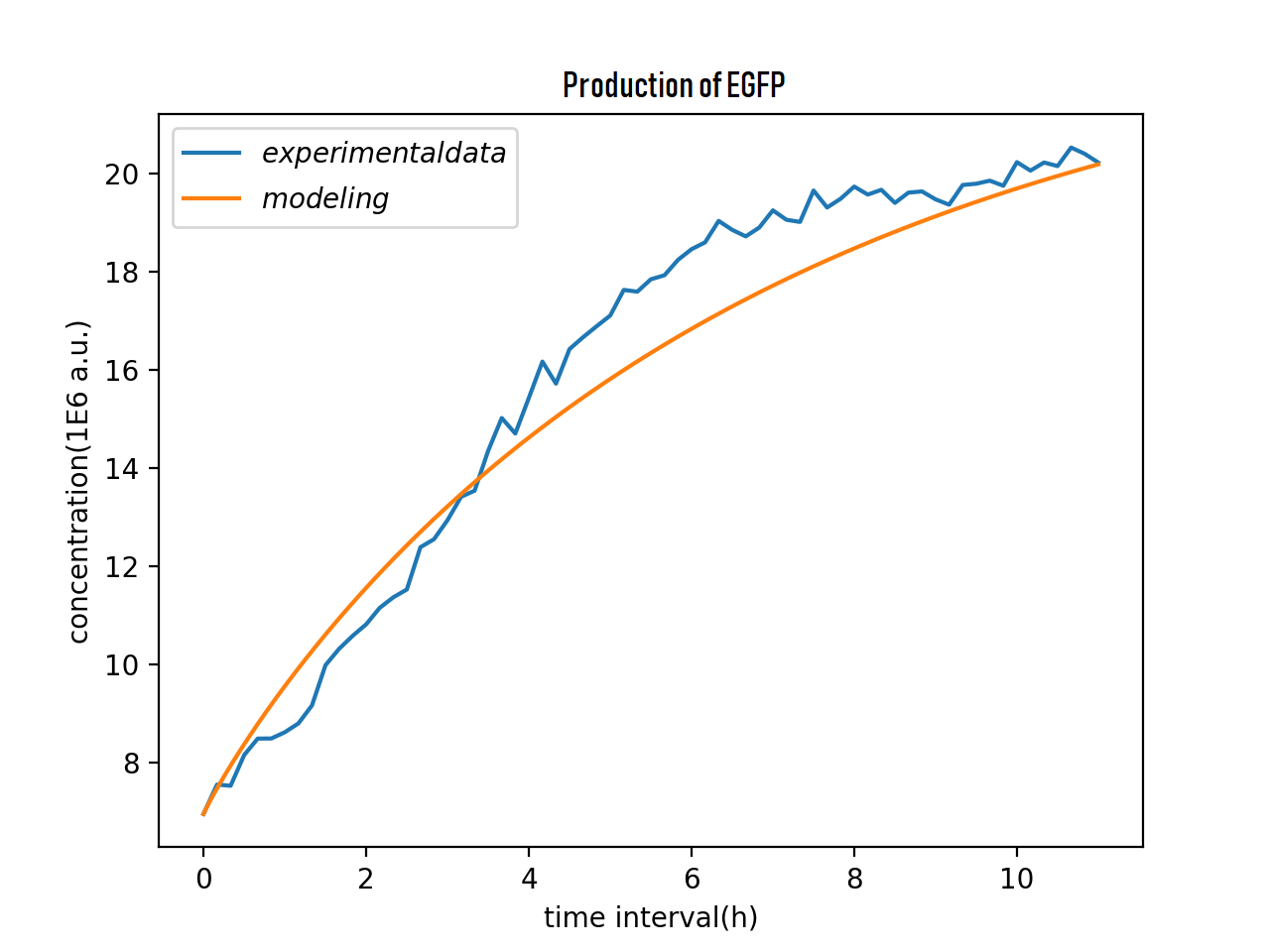From the result above, the SYSU model can prove their simulation has the same trend of the real experiment data, which also gave us a meaningful design performance forecast, which also validate our ODE model.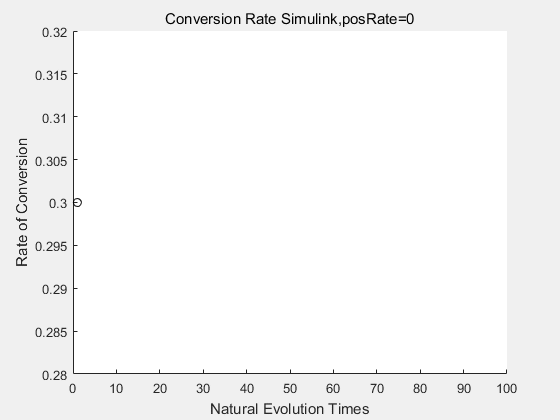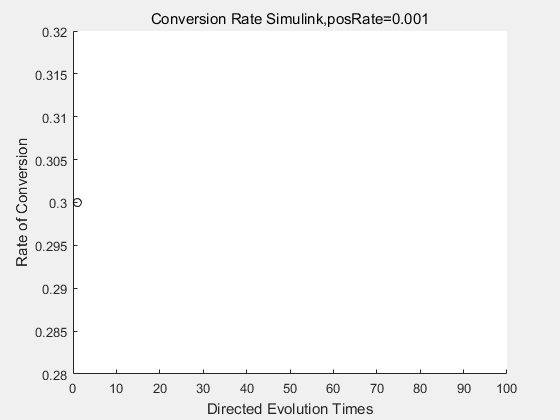With the directed evolution method to produce psicose, what matters is the conversion rate and the scale of the production system. As the results shown above, if we cultivate our system for many times, the conversion rate of DTE is increasing stably with the directed evolution method.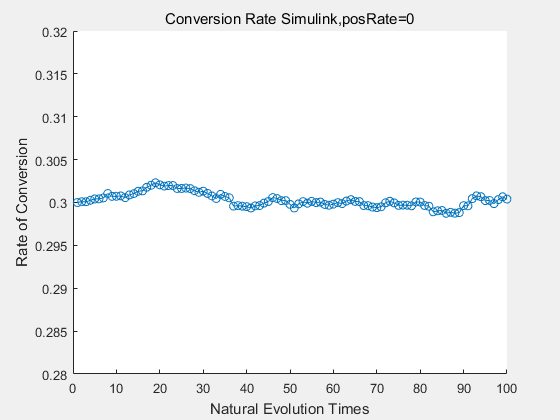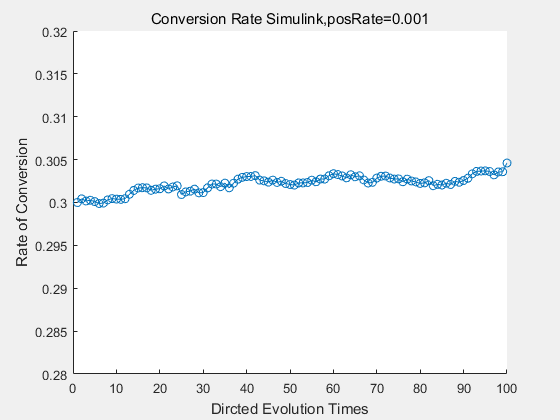If we do diretecd evolution for more times, we will get the same result and the increase of conversion rate with directed evolution is stable, while the conversion rate of DTE in natural evolution system fluctuate in a certain range.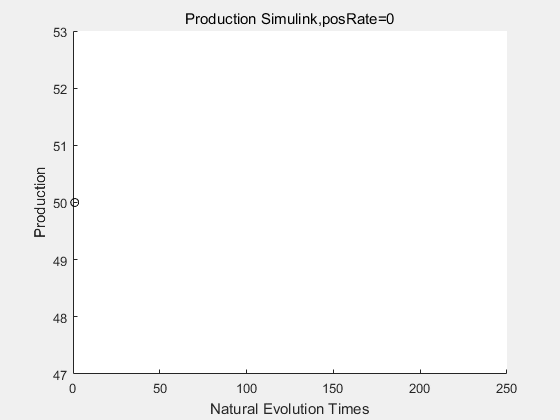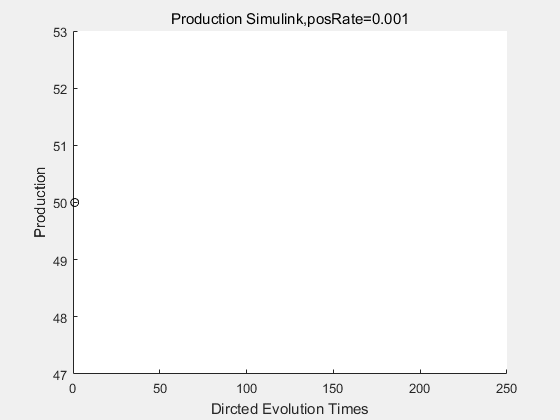If we have a system with a stable psicose production of 50,what happens in directed evolution system is the slight increase in the production with the times of producting increasing. Whereas, the production of natural evolution system is still in the original production and vibrate in a small range.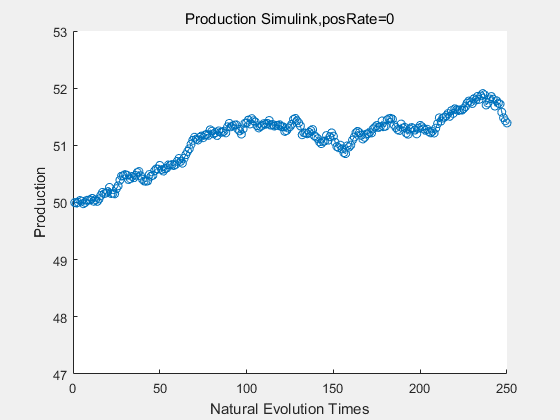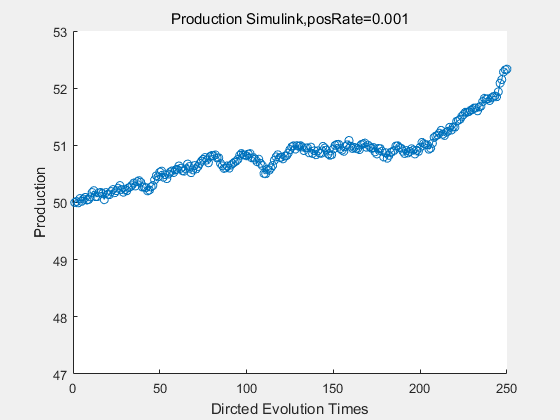With more simulations ,we can find that although the production is not always increasing all the time, the main stream of the directed evolution is to have a good production of psicose. The production of natural system will increase or decrease due to the vibration of the conversion rate, and if we select the positive strain each evolution process,, we will get the same production results which is the factory method for strain selection.